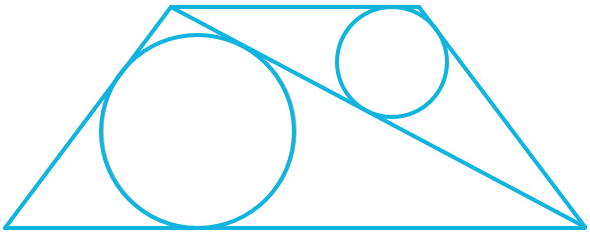# Circles Packed Into A Trapezoid

Geometry Level 5The diagram shows an isosceles trapezium divided into two triangles each inscribing a circle. Given that the length of the upper base of the trapezium is 9 and the diameters of the circles are 4 and 7, find the length of the lower base.

×